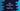# Introduction to Dart Map class# Dart Map :

Map is used to store key-value pairs in dart. Value can occur multiple times but each key must be unique. The key is used to retrieve the value associated with it. Map is growable. You can change its length.

## Map Declaration :

We can declare a map using a map constructor or using map literal.

### Using map constructor :

Using the constructor, we can create one empty Map and add key-value pairs to it :

``````main(List<string> args) {
var numberMap = Map();
numberMap = "one";
numberMap = "two";
numberMap = "three";
numberMap = "four";

print(numberMap);
}``````

It will print the below output :

``{1: one, 2: two, 3: three, 4: four}``

You can add an infinite number of key-value pairs. This approach is useful if you are adding pairs programmatically. For example, the below program will print the same output :

``````main(List<string> args) {
var number = ["one", "two", "three", "four"];
var numberMap = Map();

for (var i = 1; i < number.length+1; i++) {
numberMap[i] = number[i - 1];
}

print(numberMap);
}``````

### Using Map Literal :

For initializing a map using literal, you need to enclose the key-value pairs within a pair of curly braces :

``````main(List<string> args) {
var numberMap = {1: "one", 2: "two", 3: "three", 4: "four", 5: "five"};

print(numberMap);
}``````

It will print :

``{1: one, 2: two, 3: three, 4: four, 5: five}``

You can also create one empty map and add key-value pairs later on :

``````main(List<string> args) {
var numberMap = {};
numberMap = "one";
numberMap = "two";
numberMap = "three";
numberMap = "four";
numberMap = "five";

print(numberMap);
}``````

The output is the same as the above example.

### Create one constant map :

To create one constant or unmodifiable map, you can use the const keyword with the map literal :

``final numberMap = const {1: "one", 2: "two", 3: "three"};``

Map has a couple of different implementations like HashMap, LinkedHashMap, MapView, _etc. The iteration depends on the type of implementation. _Map constructor creates one LinkedHashMap.